Like   Tweet
 /* styles */ July 9, 2020 Dear Readers, In 2008, the third year of the Dayton Literary Peace Prize, the finalists were dominated by the voices of the young as they told stories of war, immigration, and the environment—many taking the issues into their own hands, a view well expressed in the title Break Through: Why We Can’t Leave Saving the Planet to Environmentalists. In many of the works, even the most devastating causes teach humanity, mercy, and forgiveness. One of the most poignant stories comes from a young boy whose vocal cords have been cut to assure he won’t scream if blown up as a human mine sweeper, but his night song is pure poetry; during the Civil War, a fourteen-year-old boy is sent by his mother to bring home his injured father with help from a black horse; a child in South America brings a cryptic clue to the woman who is the radio voice reaching out to the poor during a devastating war; a young immigrant from Santa Domingo struggles to find love and acceptance in pop culture America; a nine-year-old girl writes to her Japanese grandparents, survivors of the bomb in Hiroshima, to find help for her Vietnam vet father and her suicidal mother; a young Muslim in America learns to reject hatred while learning to live with religious pluralism; and two young men question the established environmental movement to find hope for the planet. In most cases, the young find hope and redemption and give the reader hope. 2008 also brought us the voices of maturity, showing that issues and politics are a passion for the older generations, too. We hear those voices questioning whether the US empire is impervious to the vices that brought down Rome. We hear a voice from the South Pacific warning us that the coral reefs that are vital to our existence are threatened with extinction, and we hear the voice of the immigrant from Haiti telling his brother that he is dying. These voices echo the young, but are now voices that realize yes, the change is up to us, but we are running out of time to make a difference. We hope these voices will be a call to action for the reader while “Expanding Your Horizons While Sheltering at Home.” Stay safe. Be well. Read books.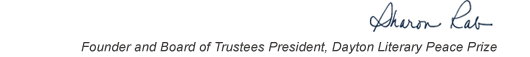table div table+table+table+table div table{width:100%;padding:0}table div table+table+table+table div table img{width:96.23%;padding:0;float:none}table div table+table+table+table div table td{width:100%;padding:0 1.88% 18px}/* styles */This list includes the winners and runners-up for 2008 and we have rich background information on each one on our website.

▪ Under Past Winners. scroll down to the year and click on it.
▪ If you click on the winners and runners-up, you will find the author’s photo and bio, a selection from the book, the judges’ citation, the writer’s reflection on literature and peace, and the option of watching the introduction and acceptance speech. If you click on the finalists, you will find a short description of each book.
 ▪ Under Past Winners. scroll down to the year and click on it.
 ▪ If you click on the winners and runners-up, you will find the author’s photo and bio, a selection from the book, the judges’ citation, the writer’s reflection on literature and peace, and the option of watching the introduction and acceptance speech. If you click on the finalists, you will find a short description of each book.

On the bottom right of the home page, you can click on COREScholar, which is built by the Chair of our Curriculum Committee Carol Loranger, Associate Dean of the College of Liberal Arts at Wright State, and maintained for us by Wright State University. There you can find interviews, TED Talks, articles, other books, films, etc. by and about each of our winning and runner-up authors. It is a great resource for students, book clubs, and readers who would like to explore an author in depth.

 table div table+table+table+table+table+table div table{width:100%;padding:0}table div table+table+table+table+table+table div table img{width:96.23%;padding:0;float:none}table div table+table+table+table+table+table div table td{width:100%;padding:0 1.88% 18px}/* styles *//* styles */ “Read global; buy local.” — Marlon James, DLPP 2009 Fiction Winner for The Book of Night Women
 table div table+table+table+table+table+table+table+table div table{width:100%;padding:0}table div table+table+table+table+table+table+table+table div table img{width:96.23%;padding:0;float:none}table div table+table+table+table+table+table+table+table div table td{width:100%;padding:0 1.88% 18px}/* styles */## 2008 Finalists - Fiction

 table div table+table+table+table+table+table+table+table+table+table div table{width:100%;padding:0}table div table+table+table+table+table+table+table+table+table+table div table img{width:96.23%;padding:0;float:none}table div table+table+table+table+table+table+table+table+table+table div table td{width:100%;padding:0 1.88% 18px}/* styles */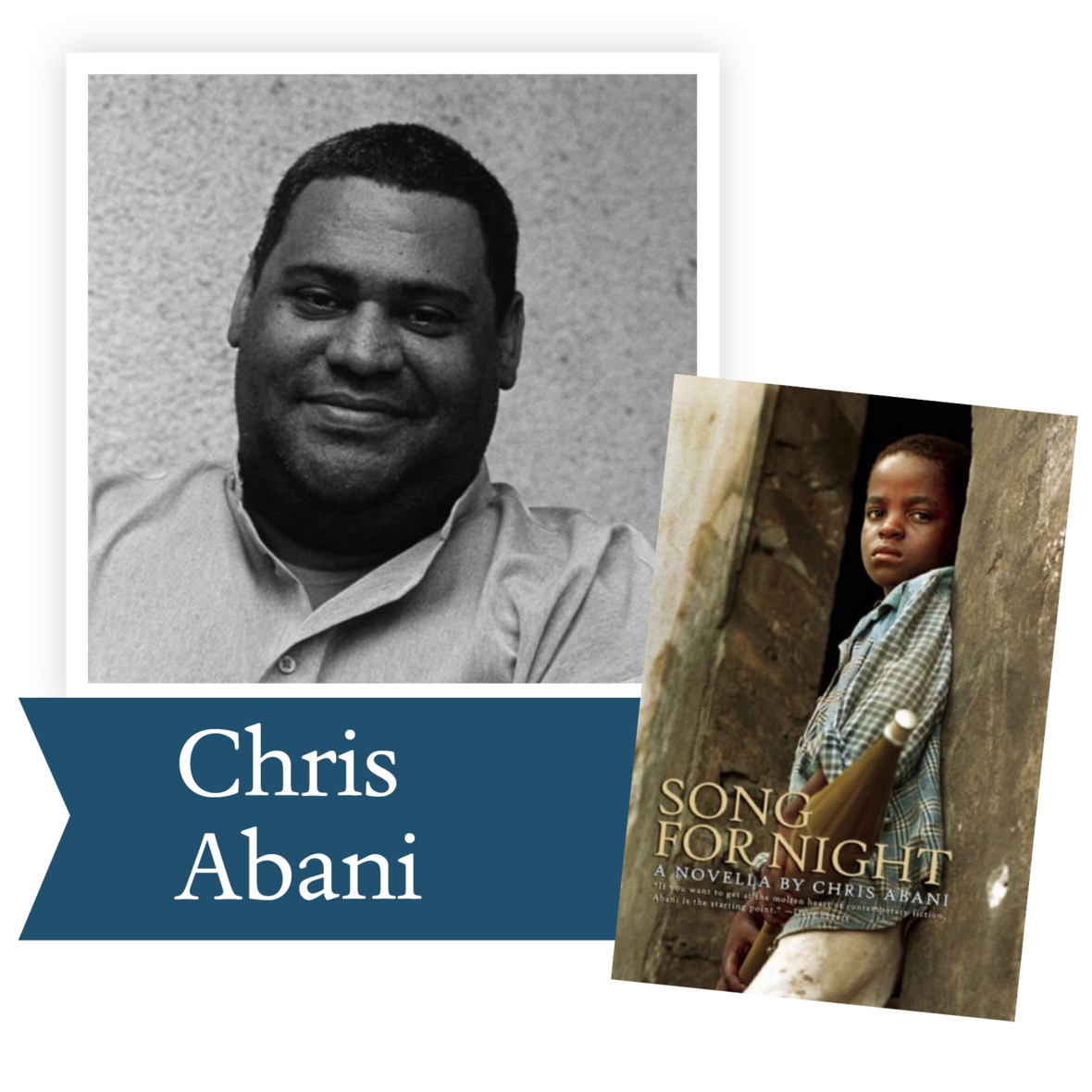Song for Night by Chris Abani (Akashic Books): Trained as a human mine detector, a West African boy soldier witnesses and takes part in unspeakable brutality.

 table div table+table+table+table+table+table+table+table+table+table+table+table div table{width:100%;padding:0}table div table+table+table+table+table+table+table+table+table+table+table+table div table img{width:96.23%;padding:0;float:none}table div table+table+table+table+table+table+table+table+table+table+table+table div table td{width:100%;padding:0 1.88% 18px}/* styles */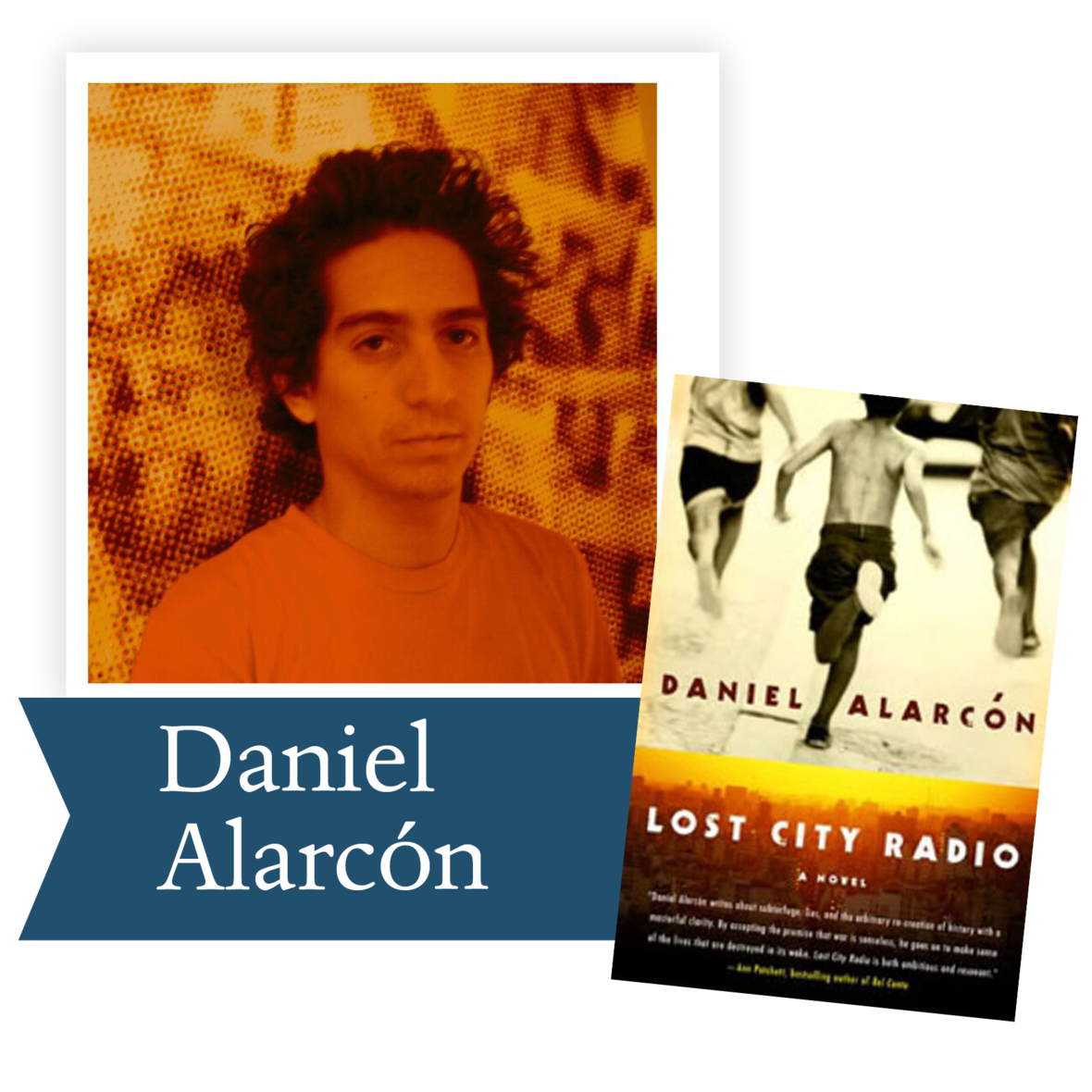Lost City Radio by Daniel Alarcón (HarperCollins Publishers): Taking place in a nameless South American country, this powerful story illustrates war's devastating impact on a society transformed by violence.

 table div table+table+table+table+table+table+table+table+table+table+table+table+table+table div table{width:100%;padding:0}table div table+table+table+table+table+table+table+table+table+table+table+table+table+table div table img{width:96.23%;padding:0;float:none}table div table+table+table+table+table+table+table+table+table+table+table+table+table+table div table td{width:100%;padding:0 1.88% 18px}/* styles */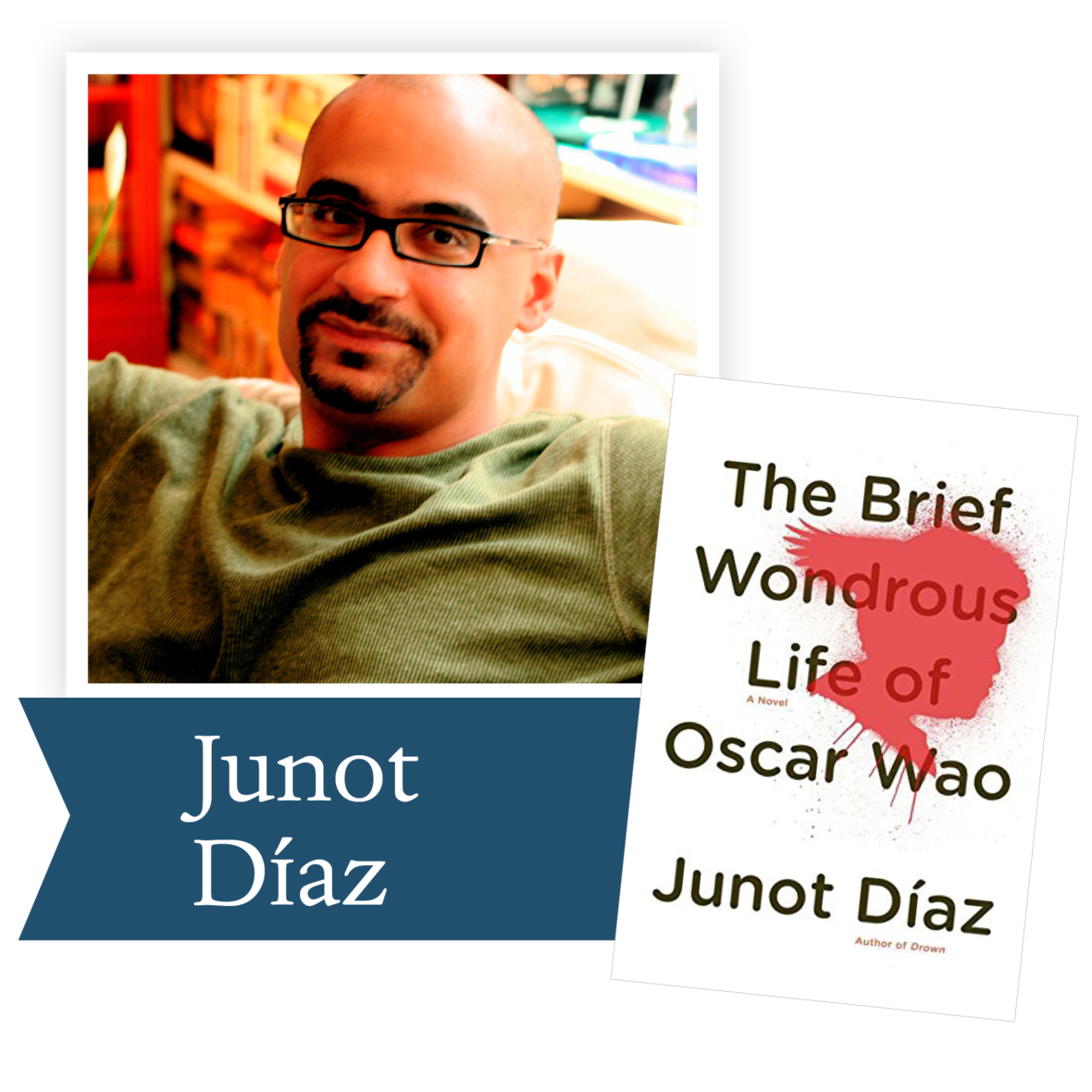The Brief Wondrous Life of Oscar Wao by Junot Díaz (Penguin Group): Mixing pop culture and political criticism, this critically acclaimed novel is a warm and humorous account of the American immigrant experience.

 table div table+table+table+table+table+table+table+table+table+table+table+table+table+table+table+table div table{width:100%;padding:0}table div table+table+table+table+table+table+table+table+table+table+table+table+table+table+table+table div table img{width:96.23%;padding:0;float:none}table div table+table+table+table+table+table+table+table+table+table+table+table+table+table+table+table div table td{width:100%;padding:0 1.88% 18px}/* styles */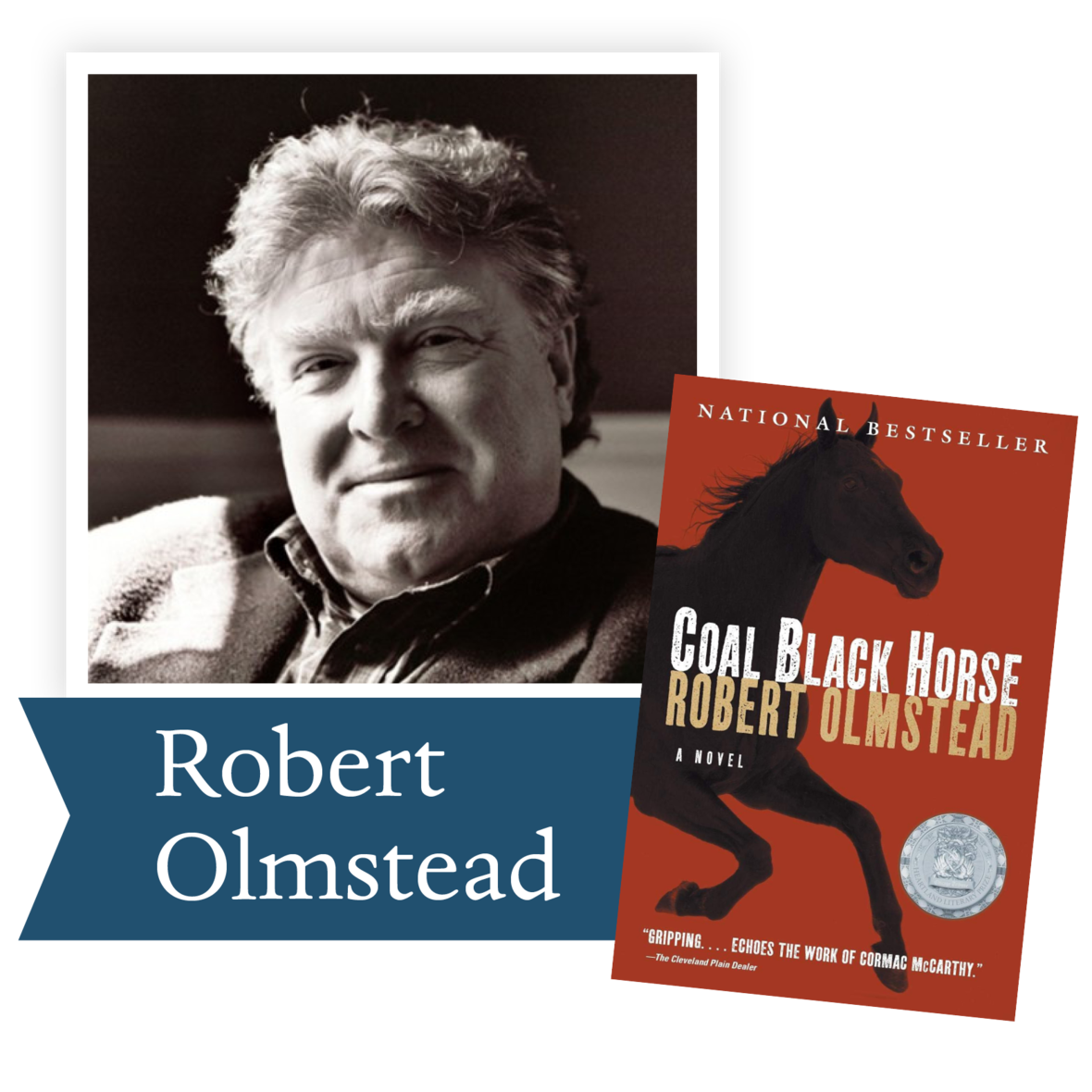Coal Black Horse by Robert Olmstead (Algonquin Books of Chapel Hill): Set during the Civil War, Coal Black Horse is a brutally honest novel depicting the horrors of war.

 table div table+table+table+table+table+table+table+table+table+table+table+table+table+table+table+table+table+table div table{width:100%;padding:0}table div table+table+table+table+table+table+table+table+table+table+table+table+table+table+table+table+table+table div table img{width:96.23%;padding:0;float:none}table div table+table+table+table+table+table+table+table+table+table+table+table+table+table+table+table+table+table div table td{width:100%;padding:0 1.88% 18px}/* styles */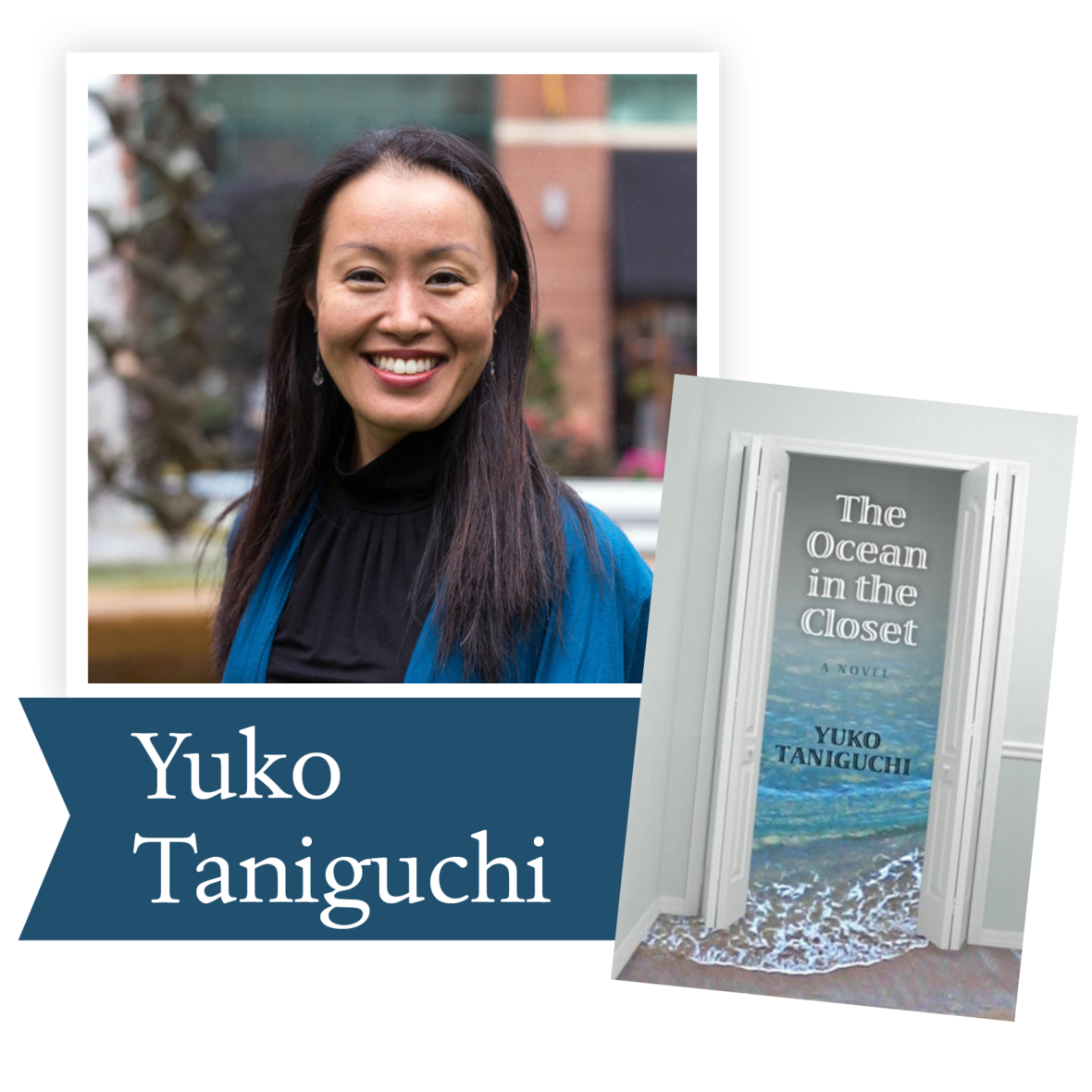The Ocean in the Closet by Yoko Taniguchi (Coffee House Press): A touching novel portraying a young woman’s journey as she helps her family heal and recover from the damages of both the Vietnam War and World War II.

 table div table+table+table+table+table+table+table+table+table+table+table+table+table+table+table+table+table+table+table+table div table{width:100%;padding:0}table div table+table+table+table+table+table+table+table+table+table+table+table+table+table+table+table+table+table+table+table div table img{width:96.23%;padding:0;float:none}table div table+table+table+table+table+table+table+table+table+table+table+table+table+table+table+table+table+table+table+table div table td{width:100%;padding:0 1.88% 18px}/* styles */## 2008 Finalists - Nonfiction

 table div table+table+table+table+table+table+table+table+table+table+table+table+table+table+table+table+table+table+table+table+table+table div table{width:100%;padding:0}table div table+table+table+table+table+table+table+table+table+table+table+table+table+table+table+table+table+table+table+table+table+table div table img{width:96.23%;padding:0;float:none}table div table+table+table+table+table+table+table+table+table+table+table+table+table+table+table+table+table+table+table+table+table+table div table td{width:100%;padding:0 1.88% 18px}/* styles */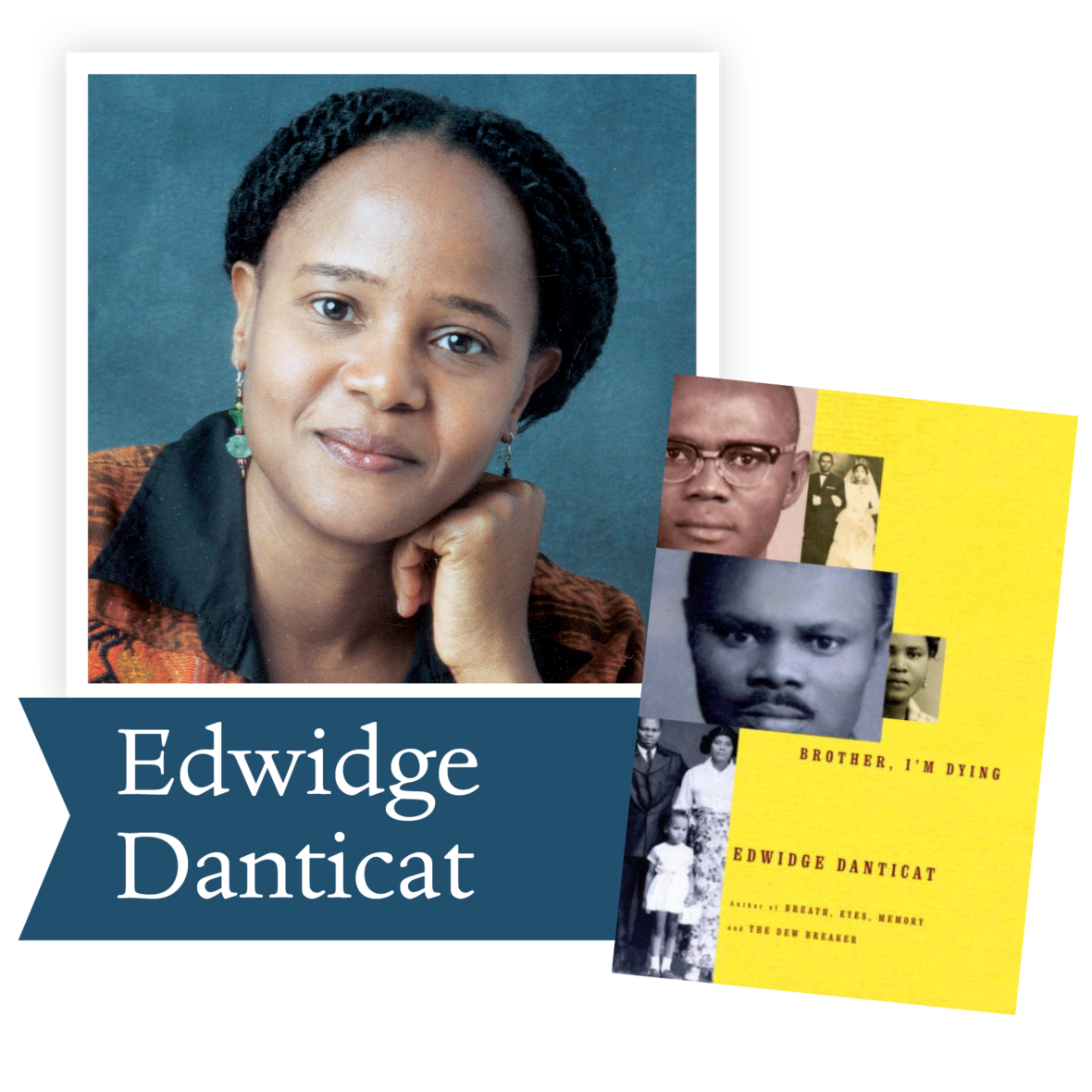Brother, I'm Dying by Edwidge Danticat (Knopf Publishing Group): A moving memoir of Danticat’s Haitian immigrant family’s struggle to stay connected in spite of living apart.

 table div table+table+table+table+table+table+table+table+table+table+table+table+table+table+table+table+table+table+table+table+table+table+table+table div table{width:100%;padding:0}table div table+table+table+table+table+table+table+table+table+table+table+table+table+table+table+table+table+table+table+table+table+table+table+table div table img{width:96.23%;padding:0;float:none}table div table+table+table+table+table+table+table+table+table+table+table+table+table+table+table+table+table+table+table+table+table+table+table+table div table td{width:100%;padding:0 1.88% 18px}/* styles */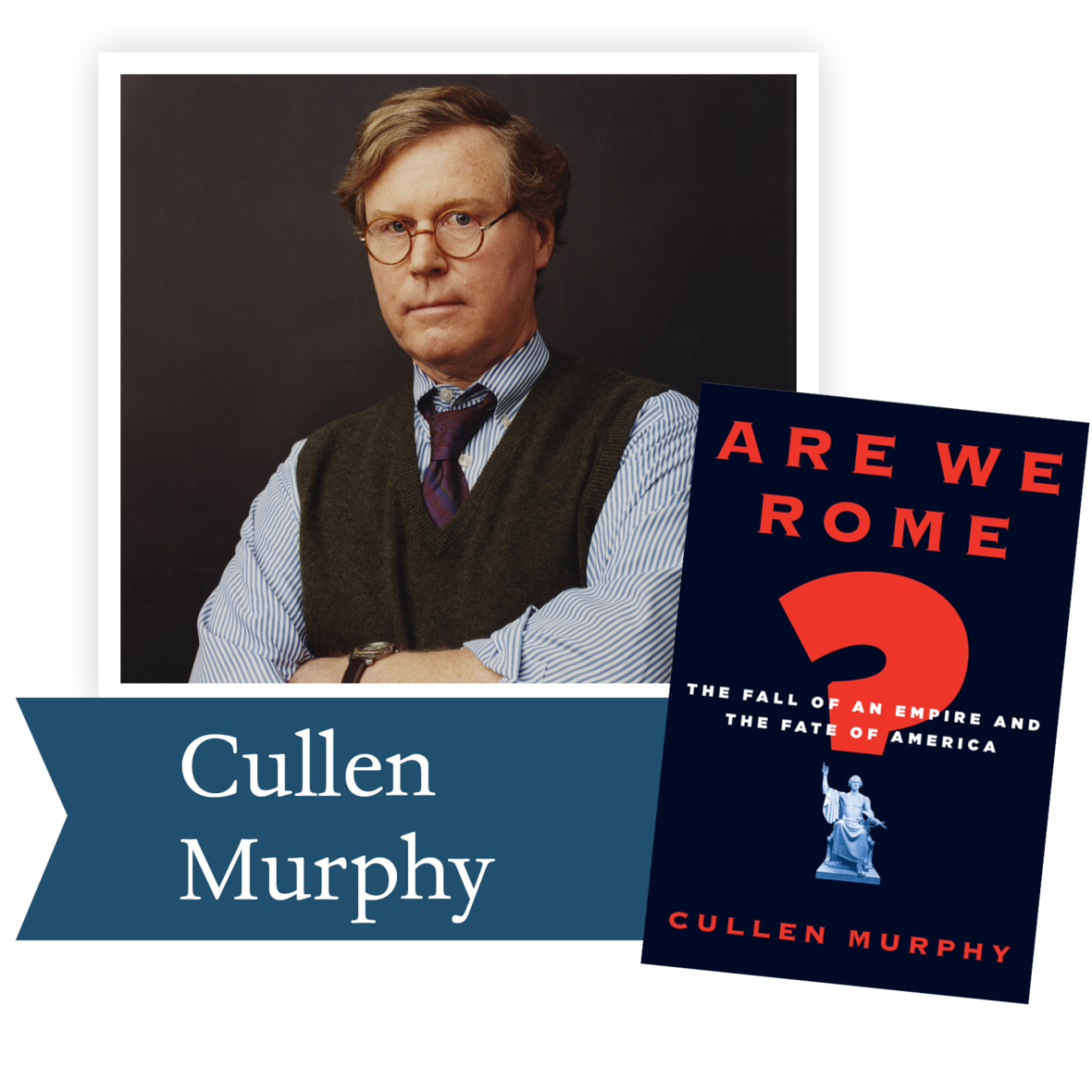Are We Rome? by Cullen Murphy (Houghton Mifflin Company): Comparing the politics and culture of Ancient Rome with that of the contemporary United States, Murphy reveals lessons on how America can avoid Rome’s demise.

 table div table+table+table+table+table+table+table+table+table+table+table+table+table+table+table+table+table+table+table+table+table+table+table+table+table+table div table{width:100%;padding:0}table div table+table+table+table+table+table+table+table+table+table+table+table+table+table+table+table+table+table+table+table+table+table+table+table+table+table div table img{width:96.23%;padding:0;float:none}table div table+table+table+table+table+table+table+table+table+table+table+table+table+table+table+table+table+table+table+table+table+table+table+table+table+table div table td{width:100%;padding:0 1.88% 18px}/* styles */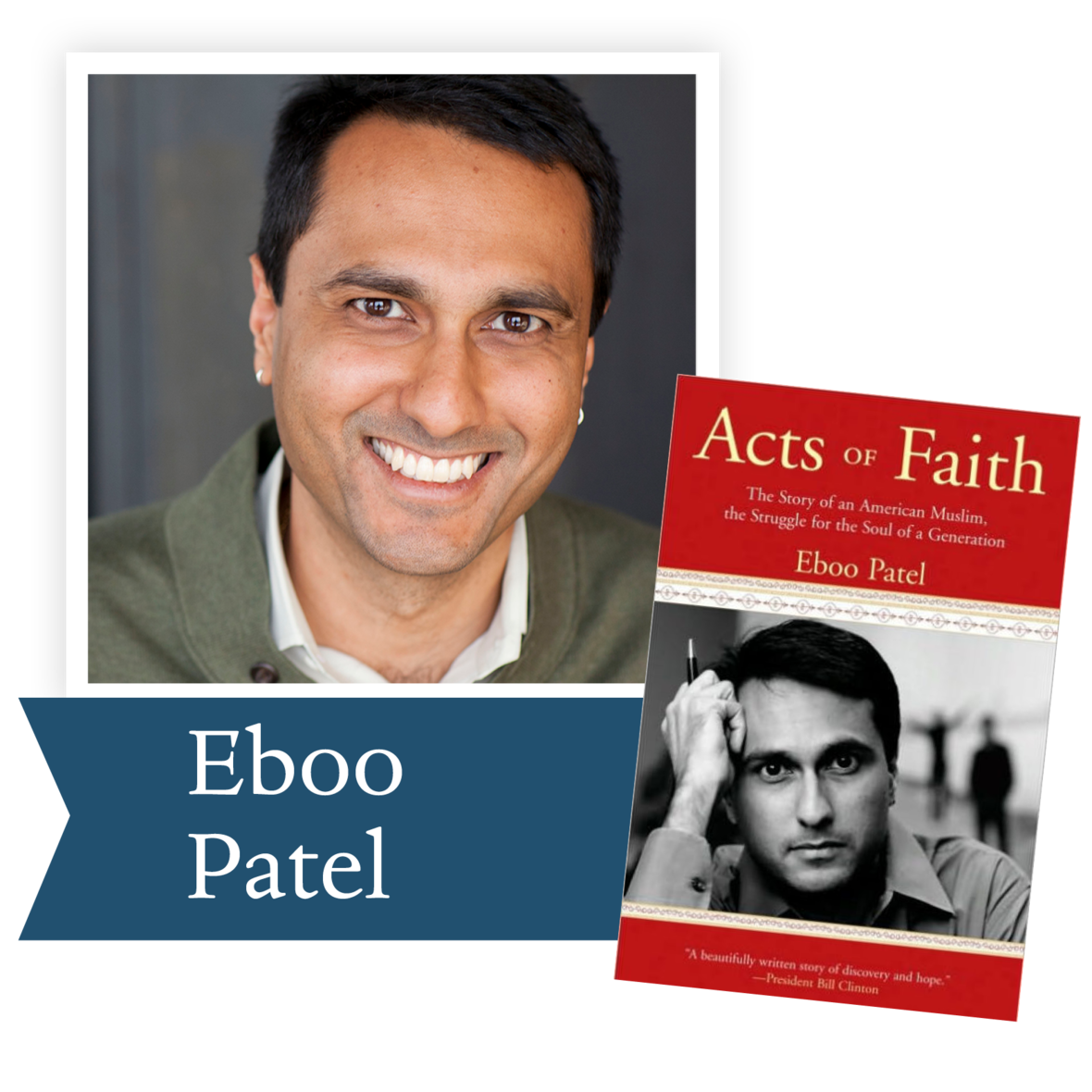Acts of Faith by Eboo Patel (Beacon Press): An American Muslim from India recounts the journey that led him to embrace religious pluralism and reject hatred.

 table div table+table+table+table+table+table+table+table+table+table+table+table+table+table+table+table+table+table+table+table+table+table+table+table+table+table+table+table div table{width:100%;padding:0}table div table+table+table+table+table+table+table+table+table+table+table+table+table+table+table+table+table+table+table+table+table+table+table+table+table+table+table+table div table img{width:96.23%;padding:0;float:none}table div table+table+table+table+table+table+table+table+table+table+table+table+table+table+table+table+table+table+table+table+table+table+table+table+table+table+table+table div table td{width:100%;padding:0 1.88% 18px}/* styles */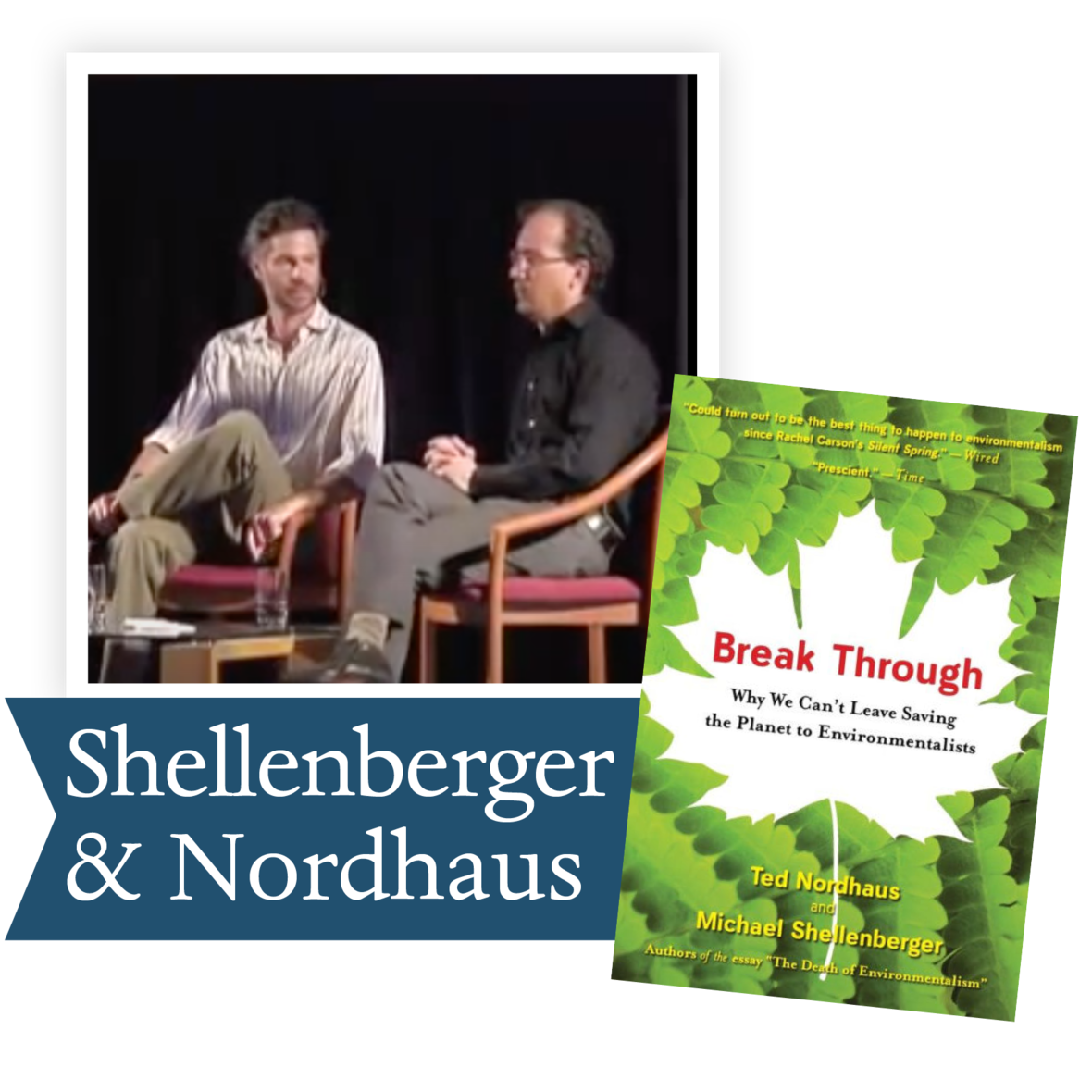Break Through by Michael Shellenberger and Ted Nordhaus (Houghton Mifflin Company): The two young environmentalists behind the controversial 2004 essay, “The Death of Environmentalism,” articulate a new politics for solving the world’s ecological crisis.

 table div table+table+table+table+table+table+table+table+table+table+table+table+table+table+table+table+table+table+table+table+table+table+table+table+table+table+table+table+table+table div table{width:100%;padding:0}table div table+table+table+table+table+table+table+table+table+table+table+table+table+table+table+table+table+table+table+table+table+table+table+table+table+table+table+table+table+table div table img{width:96.23%;padding:0;float:none}table div table+table+table+table+table+table+table+table+table+table+table+table+table+table+table+table+table+table+table+table+table+table+table+table+table+table+table+table+table+table div table td{width:100%;padding:0 1.88% 18px}/* styles */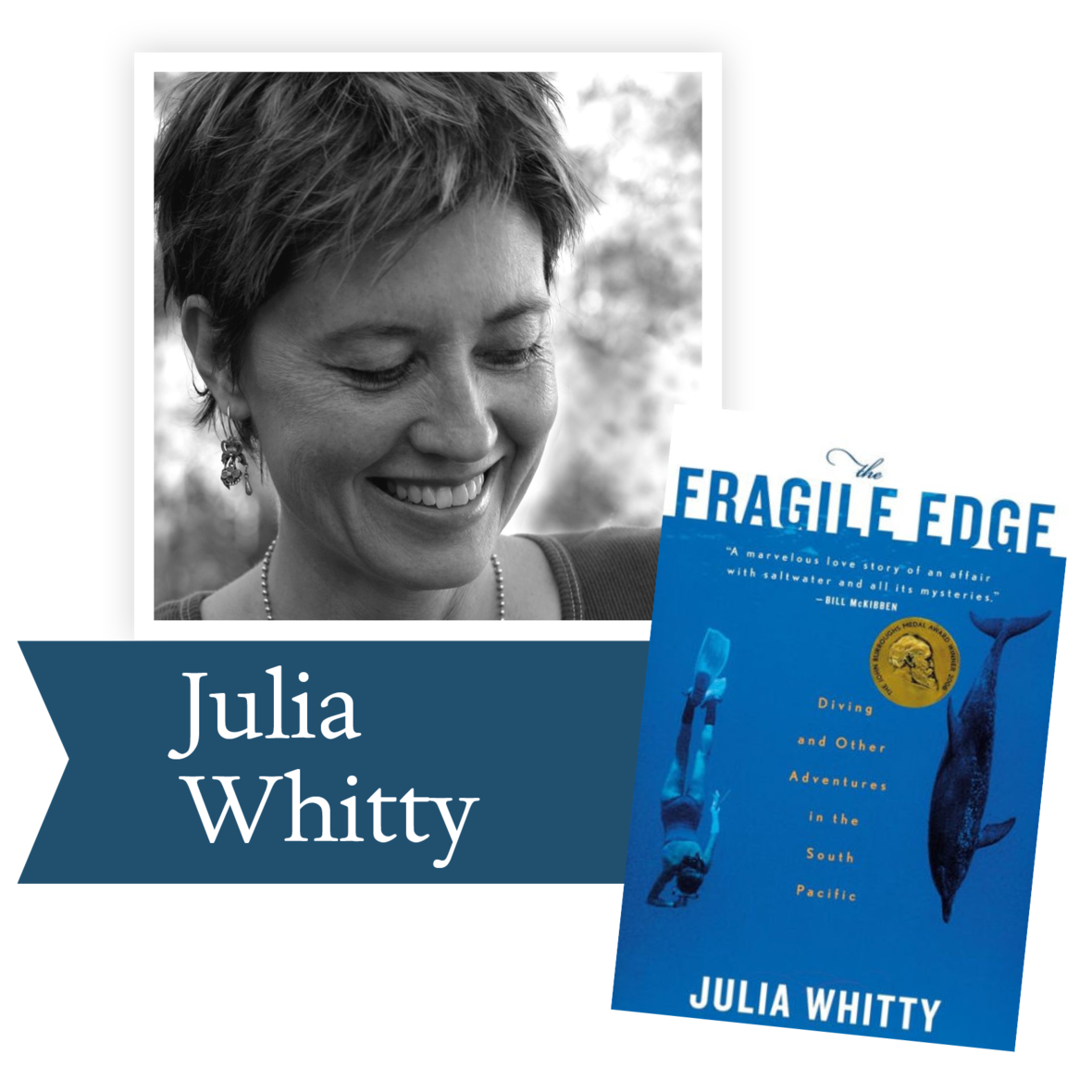Fragile Edge by Julia Whitty (Houghton Mifflin Company): Fragile Edge takes readers on an underwater journey to explore the threats facing coral reefs and why they are vital to human survival.

 table div table+table+table+table+table+table+table+table+table+table+table+table+table+table+table+table+table+table+table+table+table+table+table+table+table+table+table+table+table+table+table+table div table{width:100%;padding:0}table div table+table+table+table+table+table+table+table+table+table+table+table+table+table+table+table+table+table+table+table+table+table+table+table+table+table+table+table+table+table+table+table div table img{width:96.23%;padding:0;float:none}table div table+table+table+table+table+table+table+table+table+table+table+table+table+table+table+table+table+table+table+table+table+table+table+table+table+table+table+table+table+table+table+table div table td{width:100%;padding:0 1.88% 18px}/* styles */## 2008 Lifetime Achievement Award Winner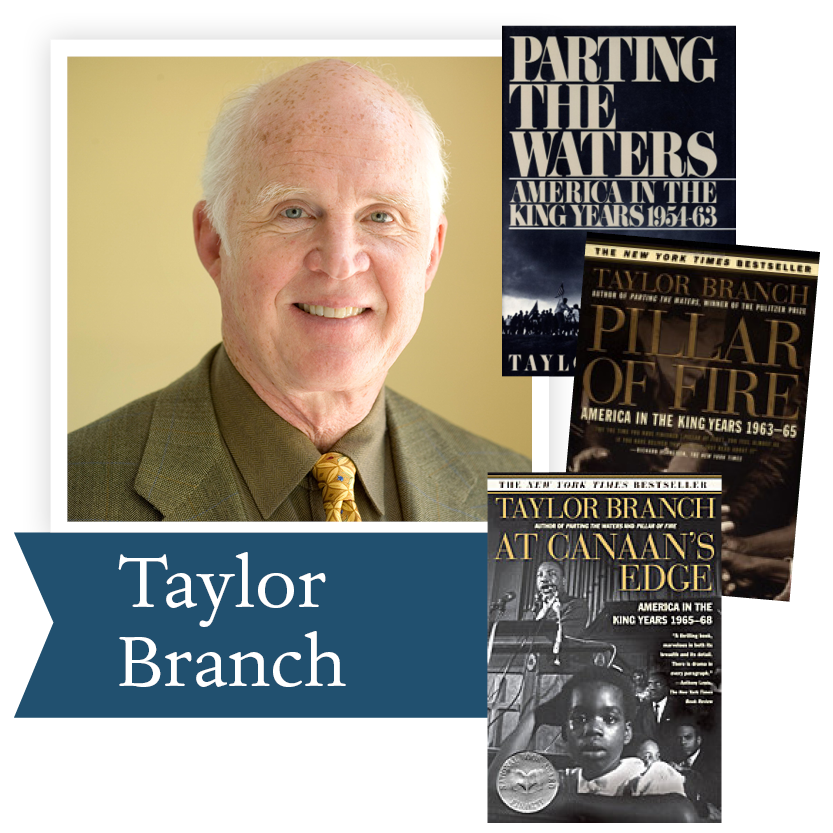Taylor Branch spent twenty-four years researching and writing the trilogy of books that have become the basis for any study of Reverend Dr. Martin Luther King, Jr. This amazing trilogy will answer many questions you might have about the history of the peace movement that we see revived throughout America and the world. The inspiration of King’s pacifism and the history of his movement for social justice and peace is reflected on our streets today.

“The crowning achievement of Branch’s King trilogy is to show anew the moral power of [nonviolent] philosophy.”
— The Atlanta Journal-Constitution

In Parting The Waters, Taylor Branch has created an unparalleled epic of America in the midst of change, poised on the threshold of its most explosive era.

In PIllar of Fire, the second volume of his three-part history, Taylor Branch portrays the Civil Rights Movement at its zenith, recounting the climactic struggles as they commanded the national stage.

At Canaan’s Edge concludes America in the King Years, a three-volume history that will endure as a masterpiece of storytelling on American race, violence, and democracy. Pulitzer Prize-winner and bestselling author Taylor Branch makes clear in this magisterial account of the civil rights movement that Martin Luther King, Jr., earned a place next to James Madison and Abraham Lincoln in the pantheon of American history.

 table div table+table+table+table+table+table+table+table+table+table+table+table+table+table+table+table+table+table+table+table+table+table+table+table+table+table+table+table+table+table+table+table+table+table+table div table{width:100%;padding:0}table div table+table+table+table+table+table+table+table+table+table+table+table+table+table+table+table+table+table+table+table+table+table+table+table+table+table+table+table+table+table+table+table+table+table+table div table img{width:96.23%;padding:0;float:none}table div table+table+table+table+table+table+table+table+table+table+table+table+table+table+table+table+table+table+table+table+table+table+table+table+table+table+table+table+table+table+table+table+table+table+table div table td{width:100%;padding:0 1.88% 18px}/* styles */table div table+table+table+table+table+table+table+table+table+table+table+table+table+table+table+table+table+table+table+table+table+table+table+table+table+table+table+table+table+table+table+table+table+table+table+table+table div table{width:100%;padding:0}table div table+table+table+table+table+table+table+table+table+table+table+table+table+table+table+table+table+table+table+table+table+table+table+table+table+table+table+table+table+table+table+table+table+table+table+table+table div table img{width:96.23%;padding:0;float:none}table div table+table+table+table+table+table+table+table+table+table+table+table+table+table+table+table+table+table+table+table+table+table+table+table+table+table+table+table+table+table+table+table+table+table+table+table+table div table td{width:100%;padding:0 1.88% 18px}/* styles */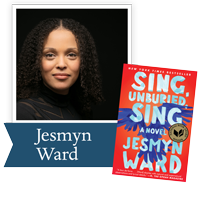Jesmyn Ward, a three-time finalist for the Dayton Literary Peace Prize, won the National Book Award for Sing, Unburied, Sing. Her work can educate us about the racial issues facing America today. Please join us in July to discuss this important book.

“The heart of Jesmyn Ward’s Sing, Unburied, Sing is story—the yearning for a narrative to help us understand ourselves, the pain of the gaps we’ll never fill, the truths that are failed by words and must be translated through ritual and song...Ward’s writing throbs with life, grief, and love, and this book is the kind that makes you ache to return to it.” —Buzzfeed

Ron Rollins, recently retired Dayton Daily News Ideas and Voices Editor, will moderate the discussion.

 table div table+table+table+table+table+table+table+table+table+table+table+table+table+table+table+table+table+table+table+table+table+table+table+table+table+table+table+table+table+table+table+table+table+table+table+table+table+table+table div table{width:100%;padding:0}table div table+table+table+table+table+table+table+table+table+table+table+table+table+table+table+table+table+table+table+table+table+table+table+table+table+table+table+table+table+table+table+table+table+table+table+table+table+table+table div table img{width:96.23%;padding:0;float:none}table div table+table+table+table+table+table+table+table+table+table+table+table+table+table+table+table+table+table+table+table+table+table+table+table+table+table+table+table+table+table+table+table+table+table+table+table+table+table+table div table td{width:100%;padding:0 1.88% 18px}/* styles */